Скачать презентацию Chapter 24 Bond Price Volatility Fabozzi Investment Management

67cdfa90fab3d9ccc8b5756922cfae36.ppt

• Количество слайдов: 22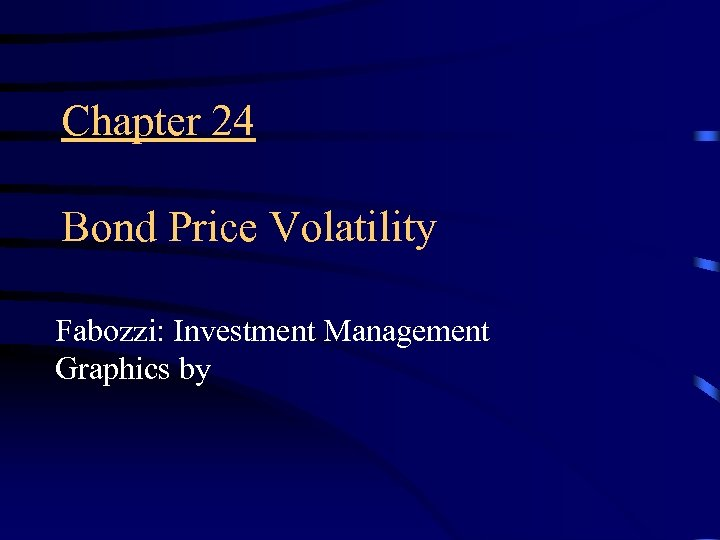Chapter 24 Bond Price Volatility Fabozzi: Investment Management Graphics by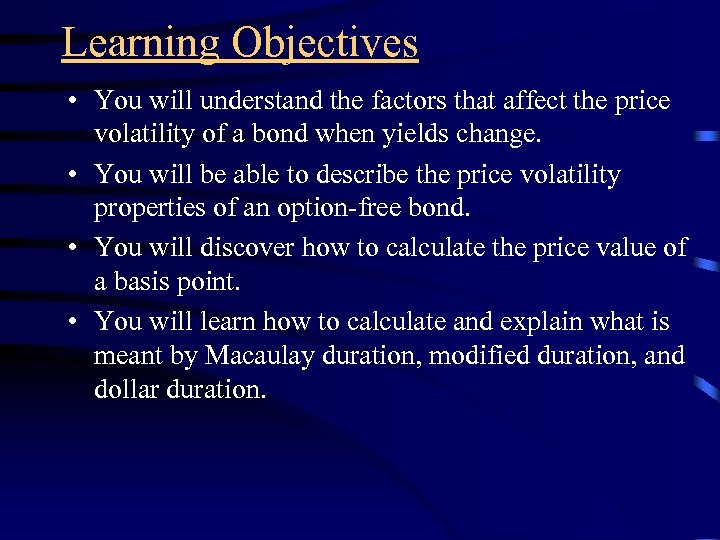Learning Objectives • You will understand the factors that affect the price volatility of a bond when yields change. • You will be able to describe the price volatility properties of an option-free bond. • You will discover how to calculate the price value of a basis point. • You will learn how to calculate and explain what is meant by Macaulay duration, modified duration, and dollar duration.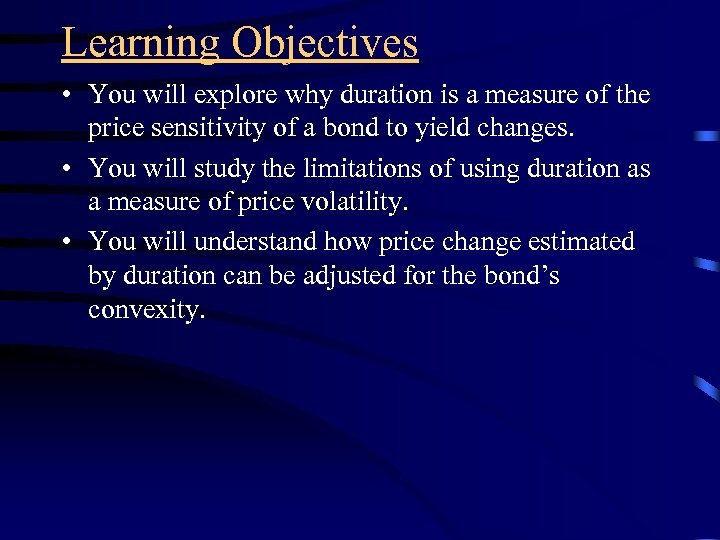Learning Objectives • You will explore why duration is a measure of the price sensitivity of a bond to yield changes. • You will study the limitations of using duration as a measure of price volatility. • You will understand how price change estimated by duration can be adjusted for the bond’s convexity.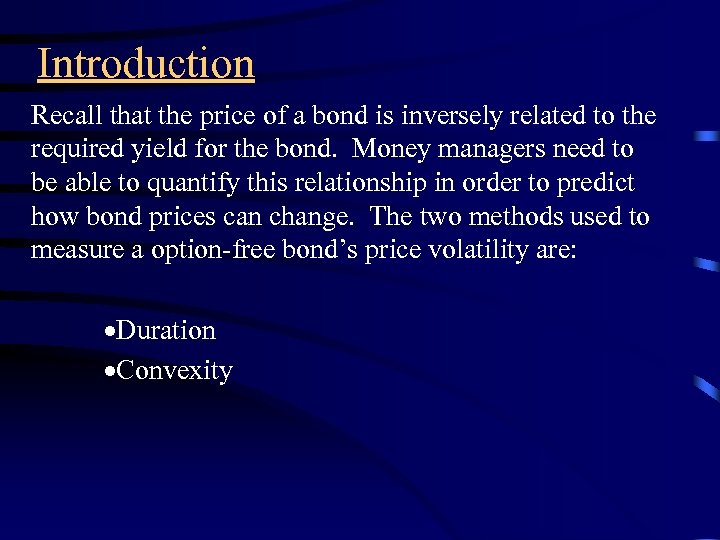Introduction Recall that the price of a bond is inversely related to the required yield for the bond. Money managers need to be able to quantify this relationship in order to predict how bond prices can change. The two methods used to measure a option-free bond’s price volatility are: ·Duration ·Convexity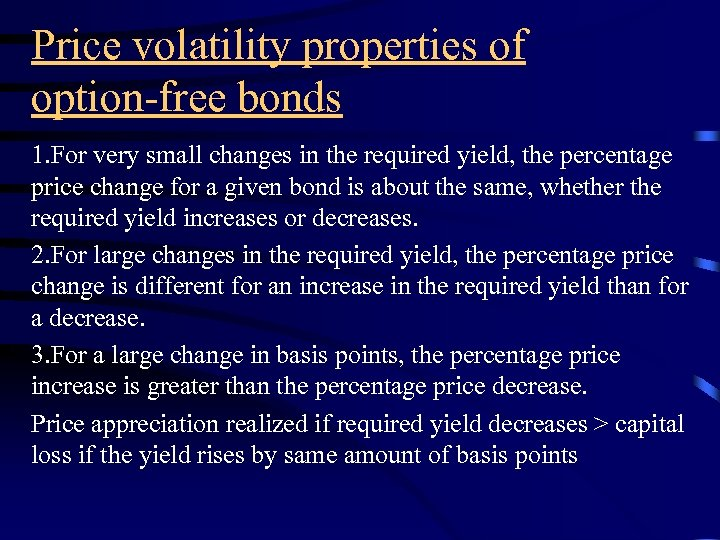Price volatility properties of option-free bonds 1. For very small changes in the required yield, the percentage price change for a given bond is about the same, whether the required yield increases or decreases. 2. For large changes in the required yield, the percentage price change is different for an increase in the required yield than for a decrease. 3. For a large change in basis points, the percentage price increase is greater than the percentage price decrease. Price appreciation realized if required yield decreases > capital loss if the yield rises by same amount of basis points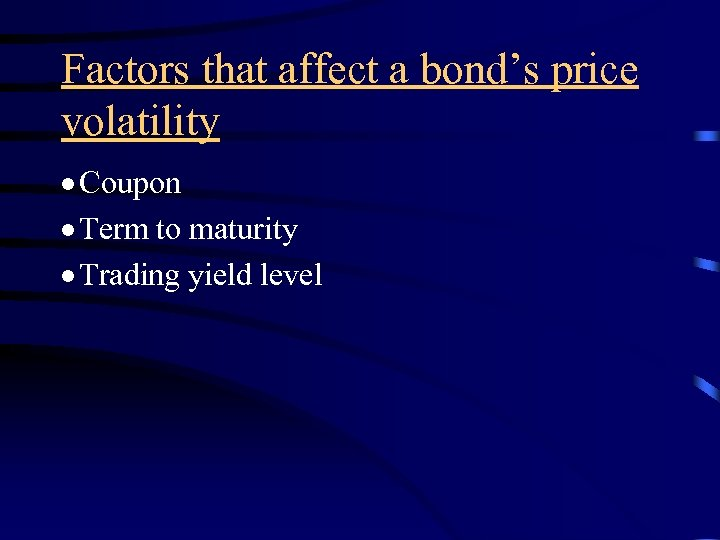Factors that affect a bond’s price volatility · Coupon · Term to maturity · Trading yield level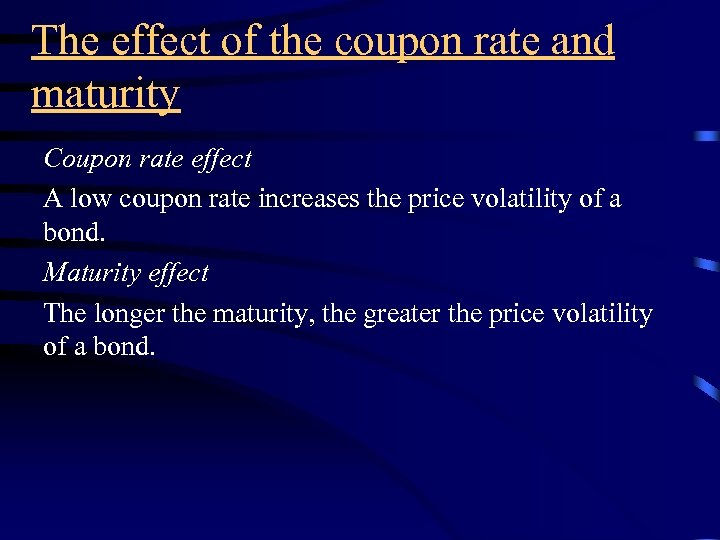The effect of the coupon rate and maturity Coupon rate effect A low coupon rate increases the price volatility of a bond. Maturity effect The longer the maturity, the greater the price volatility of a bond.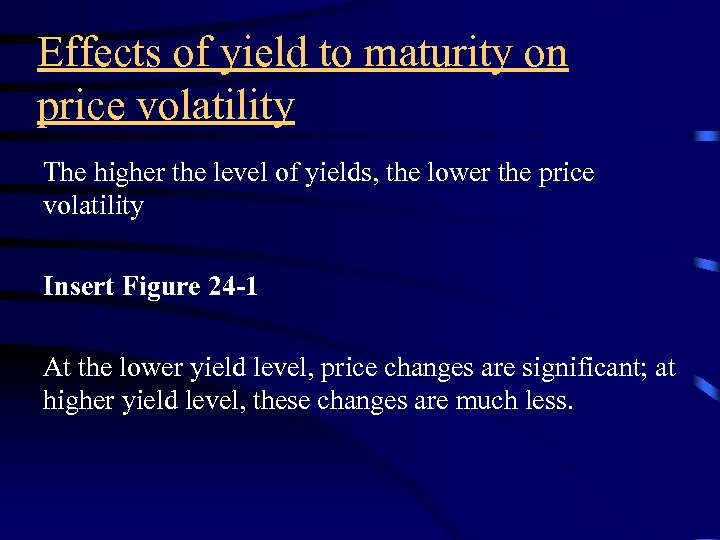Effects of yield to maturity on price volatility The higher the level of yields, the lower the price volatility Insert Figure 24 -1 At the lower yield level, price changes are significant; at higher yield level, these changes are much less.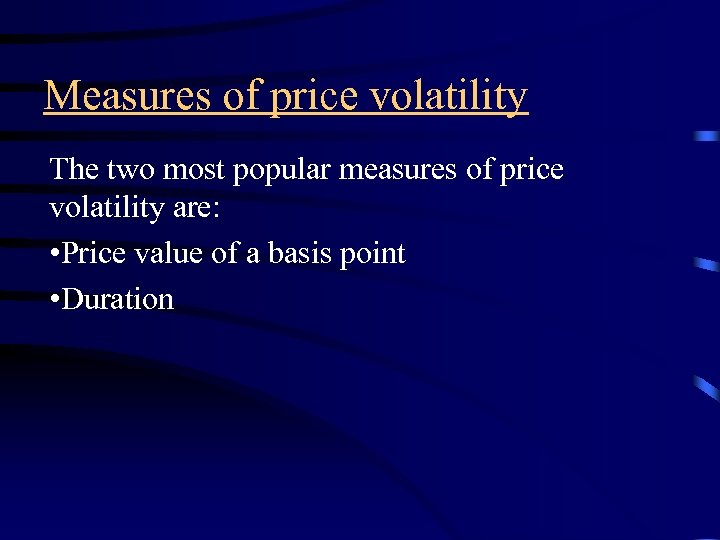Measures of price volatility The two most popular measures of price volatility are: • Price value of a basis point • Duration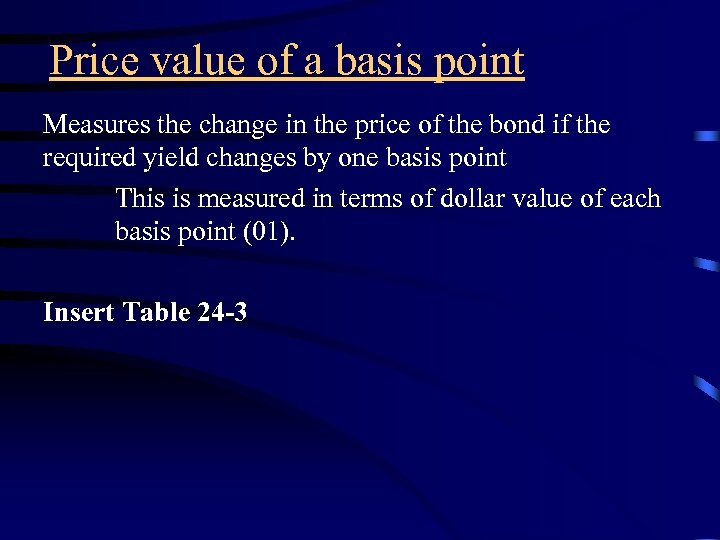Price value of a basis point Measures the change in the price of the bond if the required yield changes by one basis point This is measured in terms of dollar value of each basis point (01). Insert Table 24 -3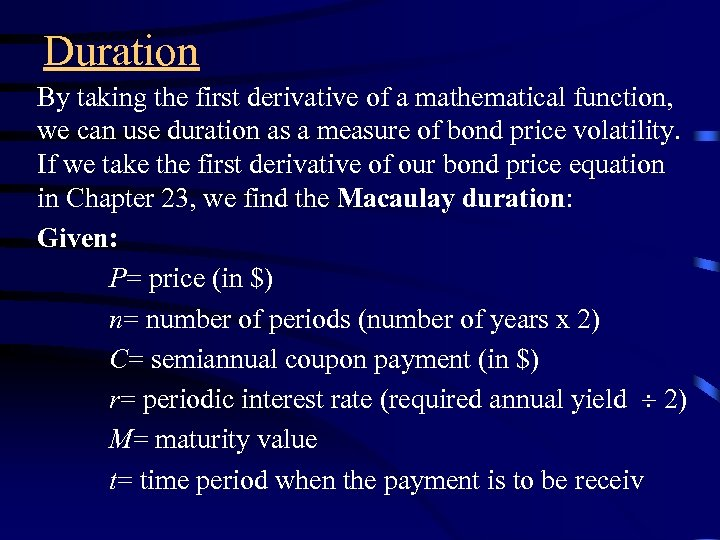Duration By taking the first derivative of a mathematical function, we can use duration as a measure of bond price volatility. If we take the first derivative of our bond price equation in Chapter 23, we find the Macaulay duration: Given: P= price (in \$) n= number of periods (number of years x 2) C= semiannual coupon payment (in \$) r= periodic interest rate (required annual yield 2) M= maturity value t= time period when the payment is to be receiv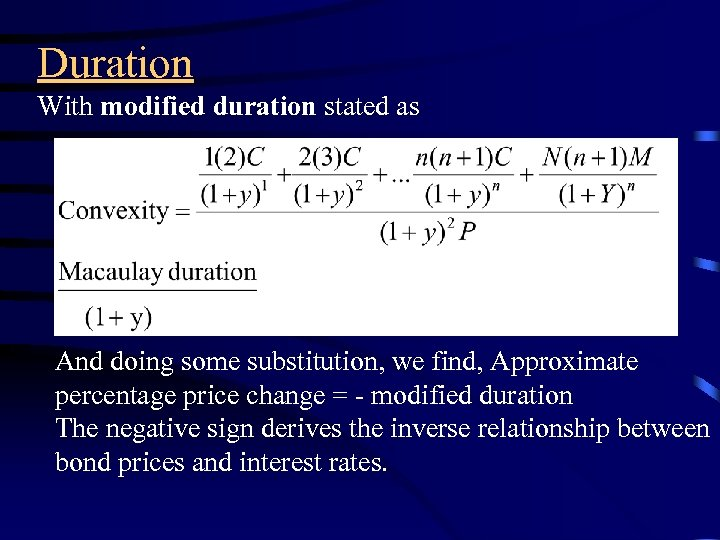Duration With modified duration stated as And doing some substitution, we find, Approximate percentage price change = - modified duration The negative sign derives the inverse relationship between bond prices and interest rates.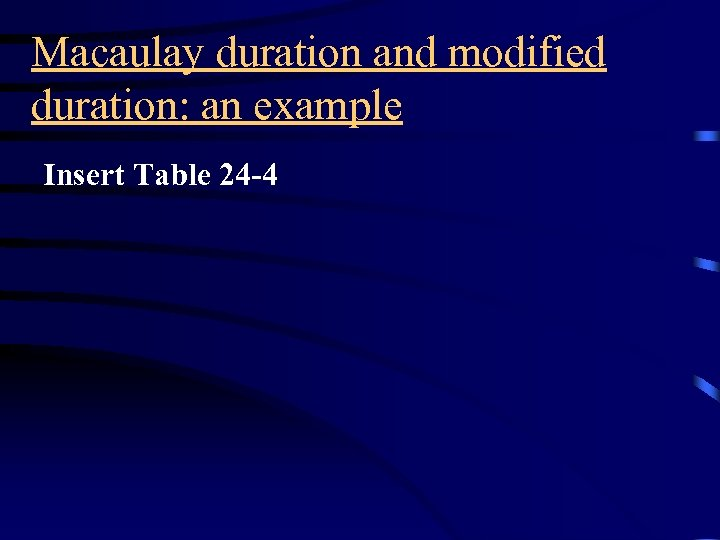Macaulay duration and modified duration: an example Insert Table 24 -4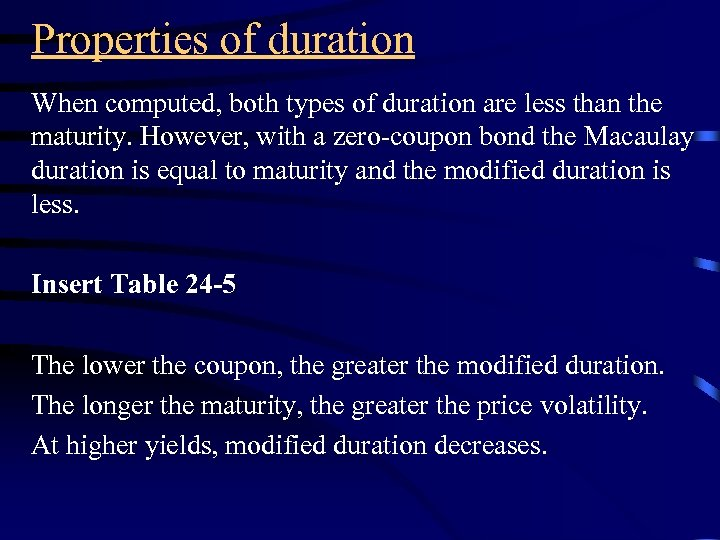Properties of duration When computed, both types of duration are less than the maturity. However, with a zero-coupon bond the Macaulay duration is equal to maturity and the modified duration is less. Insert Table 24 -5 The lower the coupon, the greater the modified duration. The longer the maturity, the greater the price volatility. At higher yields, modified duration decreases.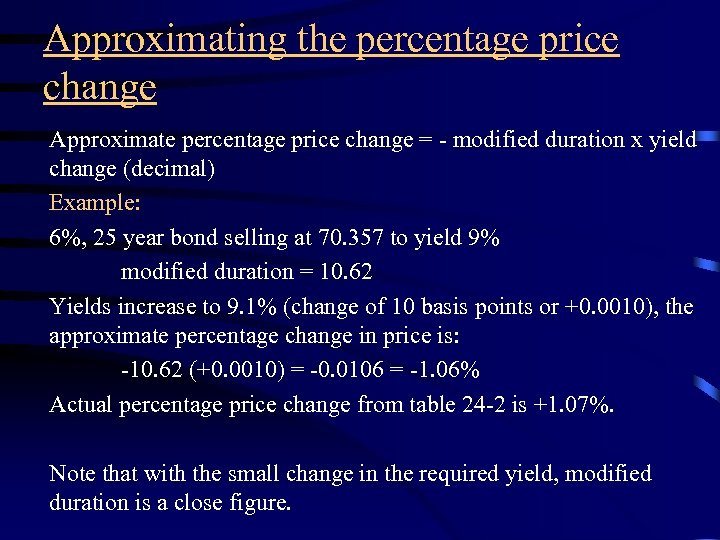Approximating the percentage price change Approximate percentage price change = - modified duration x yield change (decimal) Example: 6%, 25 year bond selling at 70. 357 to yield 9% modified duration = 10. 62 Yields increase to 9. 1% (change of 10 basis points or +0. 0010), the approximate percentage change in price is: -10. 62 (+0. 0010) = -0. 0106 = -1. 06% Actual percentage price change from table 24 -2 is +1. 07%. Note that with the small change in the required yield, modified duration is a close figure.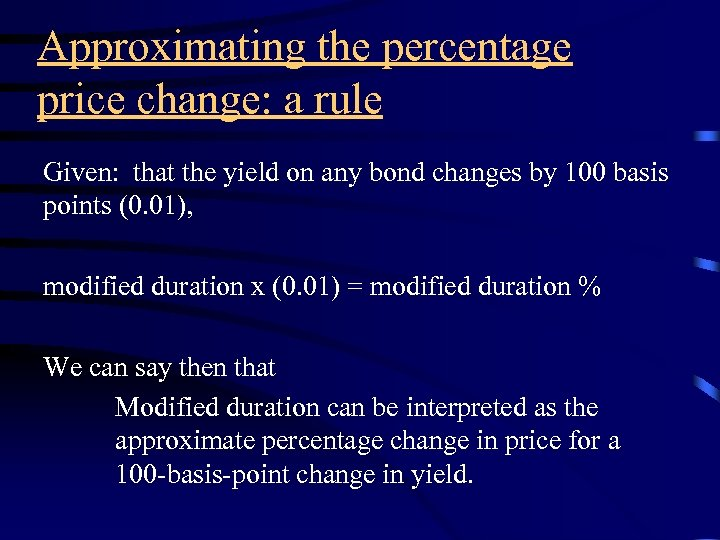Approximating the percentage price change: a rule Given: that the yield on any bond changes by 100 basis points (0. 01), modified duration x (0. 01) = modified duration % We can say then that Modified duration can be interpreted as the approximate percentage change in price for a 100 -basis-point change in yield.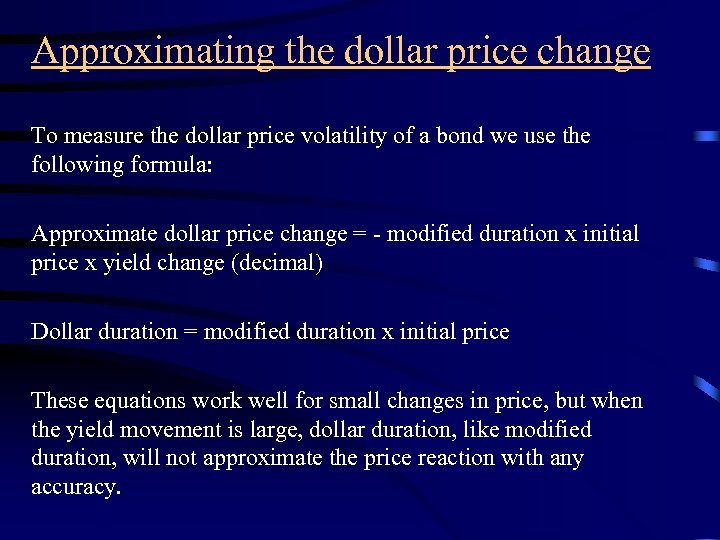Approximating the dollar price change To measure the dollar price volatility of a bond we use the following formula: Approximate dollar price change = - modified duration x initial price x yield change (decimal) Dollar duration = modified duration x initial price These equations work well for small changes in price, but when the yield movement is large, dollar duration, like modified duration, will not approximate the price reaction with any accuracy.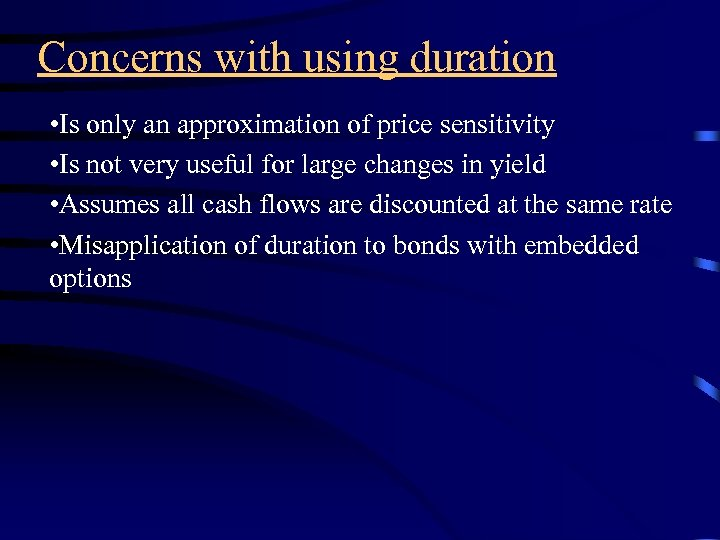Concerns with using duration • Is only an approximation of price sensitivity • Is not very useful for large changes in yield • Assumes all cash flows are discounted at the same rate • Misapplication of duration to bonds with embedded options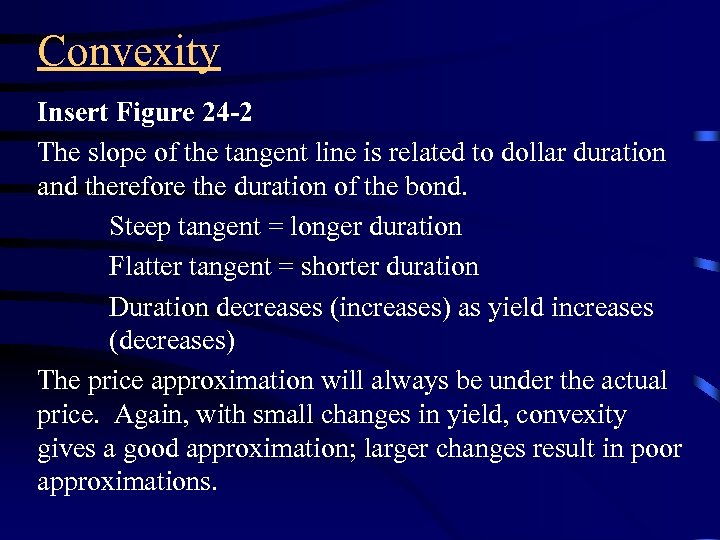Convexity Insert Figure 24 -2 The slope of the tangent line is related to dollar duration and therefore the duration of the bond. Steep tangent = longer duration Flatter tangent = shorter duration Duration decreases (increases) as yield increases (decreases) The price approximation will always be under the actual price. Again, with small changes in yield, convexity gives a good approximation; larger changes result in poor approximations.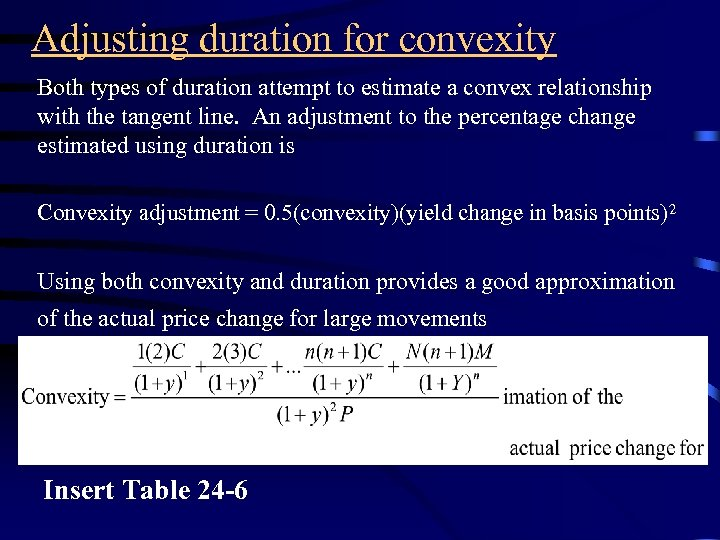Adjusting duration for convexity Both types of duration attempt to estimate a convex relationship with the tangent line. An adjustment to the percentage change estimated using duration is Convexity adjustment = 0. 5(convexity)(yield change in basis points)2 Using both convexity and duration provides a good approximation of the actual price change for large movements Insert Table 24 -6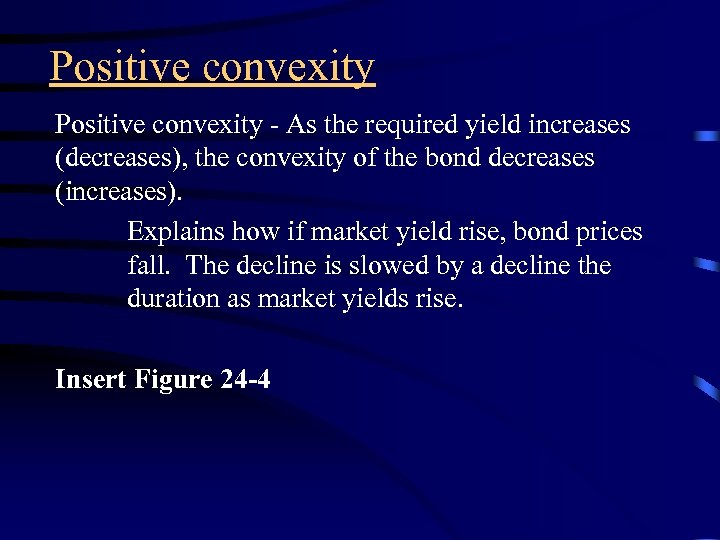Positive convexity - As the required yield increases (decreases), the convexity of the bond decreases (increases). Explains how if market yield rise, bond prices fall. The decline is slowed by a decline the duration as market yields rise. Insert Figure 24 -4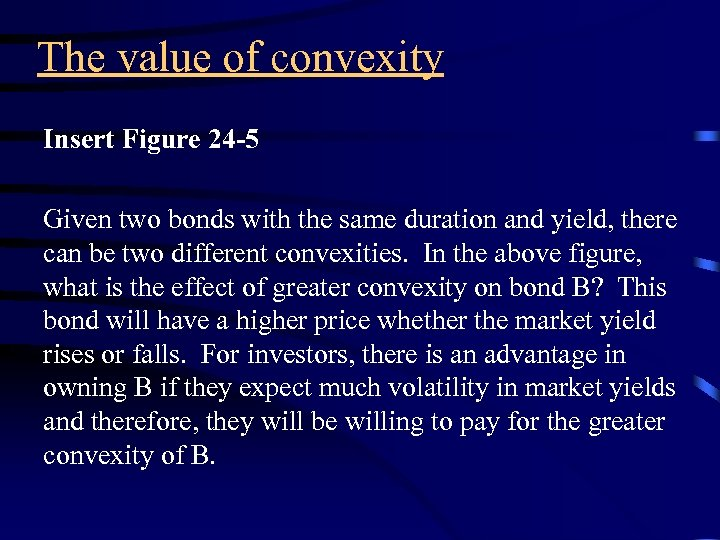The value of convexity Insert Figure 24 -5 Given two bonds with the same duration and yield, there can be two different convexities. In the above figure, what is the effect of greater convexity on bond B? This bond will have a higher price whether the market yield rises or falls. For investors, there is an advantage in owning B if they expect much volatility in market yields and therefore, they will be willing to pay for the greater convexity of B.